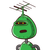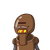# the diagonals of a rhombus are 10cm and 5.1cm . Find its area​

the diagonals of a rhombus are 10cm and 5.1cm . Find its area​

### 2 thoughts on “the diagonals of a rhombus are 10cm and 5.1cm . Find its area​”

1.$$\huge {\underline {\underline \pink{ƛƝƧƜЄƦ}}}$$

$$\bf area = \frac{1}{2} \times d1 \times d2$$

$$\bf area = \frac{1}{2} \times 10 \times 5.1$$

$$\bf area = 5 \times 5.1$$

$$\bf area = 25.5$$

2.$$area \: of \: rhombus \: = \frac{1}{2 } \times diagonal \: 1 \times diagonal2$$
$$\frac{1}{2} \times 10 \times 5.1$$
$$25.5 {cm}^{2}$$